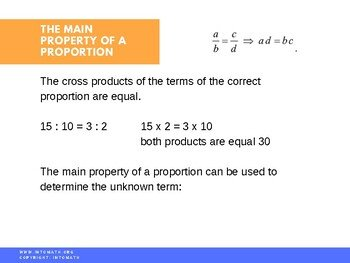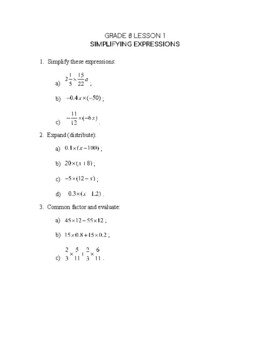CA$2.00 In this lesson you will learn about proportions, their importance, how to set them up and how to solve them. A proportion is an equivalent relationship of quantities. It is a name we give to a statement that two ratios are equal. It can be represented in two ways: two equal fractions or as a : b = c : d. We can determine whether a proportion is true or false. We can also find an unknown in a proportion or solve real life problems using proportions.Grade 8 Workbook (18 pages with answers) CA$4.00

We often use proportions to convert to or from percentages.

For example, what is 10% of 45?

We can set up a proportion: 45 corresponds to 100%, then what corresponds to 10%?

10 : 100 = n : 45

n = (45 x 10)/100

n = 4.5

There are many examples in math in which the exact answer is required.

For example, if a doctor prescribed to a patient a drug requiring a specific measure, the doctor would have to prescribe the exact amount; if the doctor simply estimated the amount, a patient could have severe side effects or it could even lead to a fatal outcome. Thus, understanding proportions could save lives!

Proportions can be solved using visual models. However, it is important to understand and learn how to quickly set them up and solve algebraically.